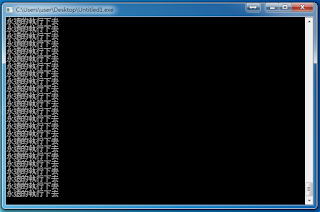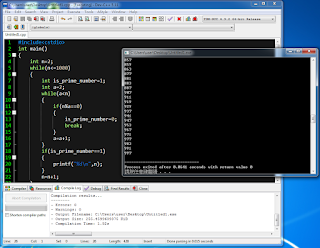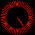## 2016年5月3日 星期二

### 給新手的C++教學 (上冊) - 5. 迴圈 (Loop)

while(繼續重複執行的條件)
{
需要被重複執行的程式碼
}

#include<cstdio>
int main()
{
int a=1;
while(a<=100)
{
a=a+1;
}
return 0;
}程式輸出「5050」了！

「=」的作用是把左邊變數的值「設定成」右邊的數字
「==」是「判斷」左邊的數字和右邊的數字有沒有相等「表單」就是外觀有設計過的程式，而不是單純的「輸入和輸出文字」相對於「表單」，這種看起來只有黑色背景、上面只會顯示文字的程式，我們稱作「終端機 (Terminal)」 這種程式比較容易製作，也就是我們現在使用C++寫的程式 當然，用C++也可以寫出「表單」

#include<cstdio>
int main()
{
int a=1,b=1;
while(a==b)
{
printf("永遠的執行下去\n");
}
return 0;
}程式會永遠的執行下去，永不停止

#include<cstdio>
int main()
{
int n;
scanf("%d",&n);
int a=1;
while(a<=n)
{
a=a+1;
}
return 0;
}

#include<cstdio>
int main()
{
int n;
scanf("%d",&n);
int factor=1;
while(factor<=n)
{
if(n%factor==0)
{
printf("%d\n",factor);
}
factor=factor+1;
}
return 0;
}

#include<cstdio>
int main()
{
int n=2;
while(n<=1000)
{
int is_prime_number=1;
int a=2;
while(a<n)
{
if(n%a==0)
{
is_prime_number=0;
break;
}
a=a+1;
}
if(is_prime_number==1)
{
printf("%d\n",n);
}
n=n+1;
}
return 0;
}好多質數XD

#include<cstdio>
int main()
{
int a=0;
while(a<=10)
{
if(a==4)
{
break;
}
a=a+1;
}
printf("%d\n",a);
return 0;
}是「4」耶！

#### 33 則留言:

1.請問找1000以內的質數我這樣寫對嗎

#include <iostream>
using namespace std;
int main(int argc, char **argv)
{
int i, j, n;

for (i = 1; i <= 1000; i++)
{
n = 0;
for (j = 1; j <= i; j++)
{
if (i % j == 0)
n++;
}
if (n == 2)
cout << i << endl;
}
}

1.哈囉～教學中已提供範例程式碼，可以比對看看您的程式碼和範例程式碼的輸出有沒有一樣哦
如果前面幾十個都對了，後面應該也沒問題～
如果真要檢查全部的數字，而不消耗眼力，是可以請電腦來幫忙的哦！
Windows有提供一個方便的指令：FC，可以用來比較兩個檔案的內容是不是一模一樣，可以跟這篇「檔案處理」所傳授的技巧配合著使用哦～

關於FC，網路上已經有諸多使用教學了，關鍵字：「windows FC 用法」

By the way並不是小莫不想幫您檢查程式碼，請見諒～與其給魚吃，不如教如何釣魚，對吧？XD有問題歡迎再問哦～

2.好喔謝謝~~

可是FC不是用來比較兩個檔案的嗎？
這樣程式碼執行要怎麼比較兩個執行檔呢？？

3.所以才說要搭配「檔案處理」的技巧呀XD
把輸出變成一個檔案~~

2.問了一個問題不見了QQ

1.3.想請問一下
我要輸入一些數字找出最大的兩數
若以三數為例
為何我如果程式沒有加註解那段
比如我依序輸入9、8、9
最大跟第二大都會是9
可是我while迴圈裡沒有判斷number=前兩項最大值的情況
意思不是指如果當number=前兩項最大值時，不會執行任何動作嗎，應該結果會跟原本前兩項做完的結果一樣不是嗎？
以下是我的code

#include <iostream>
#define N 3
using namespace std;

int main(int argc, char** argv)
{
int number;
int largest;
int secondlargest;
int counter = 3;

cout << "Please imput a number: ";
cin >> number;
largest = number;

cout << "Please imput a number: ";
cin >> number;
if (number > largest)
{
secondlargest = largest;
largest = number;
}
else
{
secondlargest = number;
}

while (counter <= N)
{
cout << "Please imput a number: ";
cin >> number;

if (number > largest)
{
secondlargest = largest;
largest = number;
}
/*else if (number == largest)
{
largest = largest;
secondlargest = secondlargest;
}*/
else if (number >= secondlargest)
secondlargest = number;

counter++;
}

cout << "\nThe largest number is: " << largest;
cout << "\nThe second largest number is: " << secondlargest;

return 0;
}

1.前面一開始第二個數似乎也是一樣
若我依序輸入9、9、8
最大跟第二大也都會是9
應該也是沒有判斷輸入=最大值的情況
所以像這種問題是都要判斷嗎？？

2.我最後把程式修改成這樣
不知道有沒有比較正確呢？

#include <iostream>
#define N 10
using namespace std;

int main(int argc, char** argv)
{
int number;
int largest;
int secondlargest;
int counter = 3;

cout << "Please imput a number: ";
cin >> number;
largest = number;

cout << "Please imput a number: ";
cin >> number;
if (number > largest)
{
secondlargest = largest;
largest = number;
}
else if (number == largest)
{
largest = largest;
secondlargest = 0;
}
else
{
secondlargest = number;
}

while (counter <= N)
{
cout << "Please imput a number: ";
cin >> number;

if (number > largest)
{
secondlargest = largest;
largest = number;
}
else if (number == largest)
{
largest = largest;
secondlargest = secondlargest;
}
else if (number >= secondlargest)
secondlargest = number;

counter++;
}

cout << "\nThe largest number is: " << largest;
cout << "\nThe second largest number is: " << secondlargest;

return 0;
}

3.如果看純看程式碼，要判斷是否正確無誤是非常困難也極具挑戰的，即使對小莫來說也一樣XD
建議想一些不同的輸入測試看看，或者在某個online judge找到對應的題目上傳您的程式碼看看有沒有AC (Accepted)，這是最快也最準確的方法之一哦～
目測您的程式碼應該是OK的，不過不保證XD

4.恩恩好的~~
那我的問題是想問說

如果我沒有判斷 輸入是否=最大值
那輸入剛好=最大值時
不是應該會沒有執行任何動作嗎
(也就是維持前一動作設定好的最大值與第二大值)
還是我的理解錯誤了呢？？

5.看您的「第二大值」的定義囉～
如果是指數值上第二大，那不用執行任何動作沒錯
如果是指挑出第二大的數字，那就是最大值有兩個就會讓最大和第二大相同囉(雖然數值相同，但因為是兩個數字，所以兩個都要算)
依您的字面意思+小莫的個人主觀認定，應該是前者，不過每個人的理解都會有落差，應該說清楚、講明白

6.恩恩是的
比如說輸入9、9、8
我是要挑出最大=9，第二大=8
但是我的問題是
如果依照我最開始的程式(亦即沒有判斷輸入是否等於最大值時)
最大跟第二大會變成都是9

而我是想問
如果我沒有判斷 輸入是否=最大值
那輸入剛好=最大值時
不是應該會沒有執行任何動作嗎
(因為我程式沒有寫到這個條件)

所以也就是維持前一動作設定好的最大值與第二大值
(亦即輸入此數等同於沒輸入的意思)
(舉例:輸入9、8、9、7，在第三次輸入第二個9時，因為沒有寫判斷輸入是否等於最大值，而剛好輸入等於最大值，因此不執行任何動作，維持第二大值為8)
(亦即「不需」寫入判斷相等的code)

但是事實是跑程式時
若沒有寫判斷相等的code
會錯誤

這是為什麼呢？
是我把C語言的邏輯理解錯誤了嗎？

7.不知道您懂不懂我的意思呢QQ

8.9.請問您有沒有嘗試過到處printf(或cout)看看哪一段程式碼被執行到呢？

10.也許會試出意料之外的結果哦～(?)XD

4.網誌管理員已經移除這則留言。

5.這是我打的程式碼
我想列出99乘法

#include<cstdio>
int main()
{
printf("請輸入一個值(1~9),本程式會列出它的九九乘法");
int b;
scanf("%d\n",&b);
int a=1;
while(a<=9)
{
printf("%d\n",a*b);
++a;
}
return 0;
}

請問為什麼要輸入2次才能運作?
而且第2次輸入的值好像不影響答案?

1.嗨嗨您好
您的scanf裡面多了一個\n哦～ ^_^

6.Thanks!
Sorry現在才看到

1.恩恩不會，有需要歡迎隨時再來留言哦XD

7.為甚麼會有沒有輸出的情況??求解
#include<iostream>
using namespace std;
int main(){
int n,i,a=1;
while(cin>>n){
for(i=2;i<=n;i++){
if(n%i==0){
for(int j=2;j<=i;j++){
if(i%j==0){
a=0;
break;
}
}

}
}
if(a==1)cout<<i<<endl;
}
}

1.您的cout前面有個if哦~
當a不等於1的時候，cout就不會執行，自然就不會有輸出了XD

8.請問n%factor是甚麼意思？看了幾次都不明白

1.n%factor 就是 「n / factor的餘數」，eg. 5%3就是2，5%4是1，5%5是0。
上一章 有補充說明喔~~
https://codingsimplifylife.blogspot.com/2016/05/c-4-process-control.html

2.謝謝 @鯤 的說明，很完整！
那小莫就不用補充囉XD

9.請問為什麼這個無法跑出答案呢?
#include
int main()
{
int n=1000;
while(n<=1)
{
if(n%2==0||n%3==0||n%5==0||n%7==0||n%11==0||n%13==0||n%17==0||n%19==0||n%23==0||n%29==0||n%31==0)
{
n=n-1;
}
else
{
printf("%d",n);
n=n-1;
}
}
return 0;
}

1.當n等於2的時候會進到if而不是else哦，因為2%2==0，您可能要再多做一些判斷？

10.請問如何用4個迴圈寫以下的程式?
1
12
123
1234
12345
5
44
333
2222
11111

1.int n,j;
for(n=1;n<=5;n++)
{
for(j=1;j<=n;j++);
printf("*");
printf("\n");
}

return 0;

2.哈哈哈這要請您自行思考囉，這才是寫程式最好玩的地方哪XD

11.請問本篇的第一個例子中的：